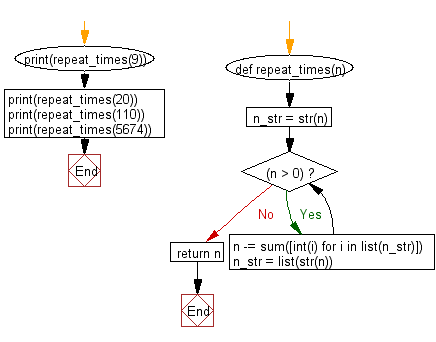﻿ Python: Accept a positive number and subtract from this number the sum of its digits - w3resource# Python: Accept a positive number and subtract from this number the sum of its digits

## Python Basic - 1: Exercise-23 with Solution

Write a Python program that accept a positive number and subtract from this number the sum of its digits and so on. Continues this operation until the number is positive.

Sample Solution:

Python Code:

``````def repeat_times(n):
s = 0
n_str = str(n)
while (n > 0):
n -= sum([int(i) for i in list(n_str)])
n_str = list(str(n))
s += 1
return s
print(repeat_times(9))
print(repeat_times(21))
``````

Sample Output:

```1
3
```

Flowchart:## Visualize Python code execution:

The following tool visualize what the computer is doing step-by-step as it executes the said program:

Python Code Editor:

Have another way to solve this solution? Contribute your code (and comments) through Disqus.

What is the difficulty level of this exercise?

Test your Python skills with w3resource's quiz

﻿

## Python: Tips of the Day

Python: The Zip() Function

```>>> students = ('John', 'Mary', 'Mike')
>>> ages = (15, 17, 16)
>>> scores = (90, 88, 82, 17, 14)
>>> for student, age, score in zip(students, ages, scores):
...     print(f'{student}, age: {age}, score: {score}')
...
John, age: 15, score: 90
Mary, age: 17, score: 88
Mike, age: 16, score: 82
>>> zipped = zip(students, ages, scores)
>>> a, b, c = zip(*zipped)
>>> print(b)
(15, 17, 16)
```